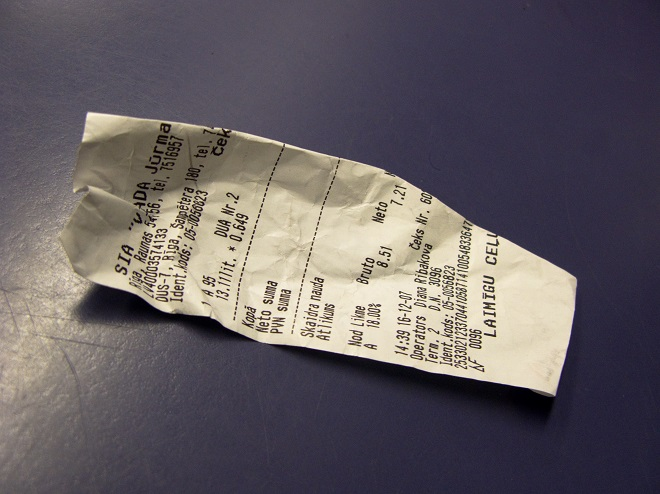# Stained billThe following note was found while leafing through the deceased merchant's notebook:

"From the sale. . . cloth remnants after 49 PLN 36 gr for each received * * * 7 PLN 28 gr“.

This note was drenched in ink in some places like this, that neither the number of units sold could be deciphered,, or the first three digits of the sum received. Will it be possible to reconstruct the number of pieces of cloth sold and the total amount obtained from this sale on the basis of known items??

The task can be solved this way:

According to the data, the total amount obtained does not exceed, of course 10 000 zlotys. So the number of leftovers sold is not greater than 203.

The last digit of an unknown number of scraps should be like this, so that when you multiply by 6 gave a product ending with 8; such a number can only be 3 or 8.

Suppose, that the last digit of an unknown number of remnants was 3. The value of the three leftovers would be 14 808 pennies. Subtracting this number from the sum obtained, we get a number ending with 920.

Second last digit, that is, the tens digit in the number of leftovers can be either 2, or 7, because only these numbers, multiplied by 6 "will give a product ending in 2.

Let's assume, that an unknown number ends with 23. By subtracting the price 23 pieces of cloth from the total sum obtained from the sale we will get a number ending with 200. The third digit could be either 2, or 7; but because of, that the unknown number does not exceed 203, our supposition should be rejected.

If we accept, that an unknown number ends with 73, then the third digit would be either 4, or 9; such a supposition must also fall away.

So the last digit can't be 3. A guess remains, that it is 8. They will show us reasoning similar to the previous ones, that the second digit could be either 4, or 9; of the two assumptions only the latter is plausible.

The task has only one solution: the leftover cloth was sold 98, the total sum obtained is 4837 PLN 28 gr.

Of course, problems of this type can also be solved algebraically.